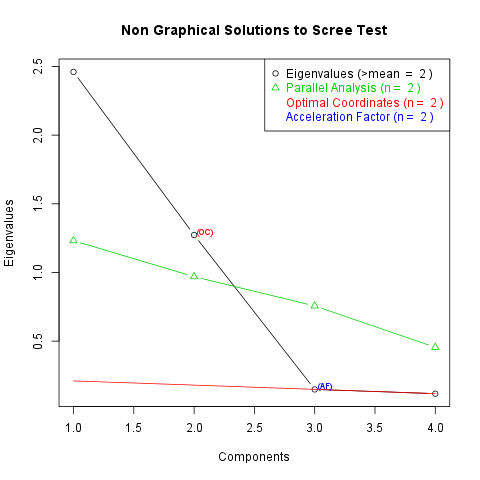## Description

In this template Rapporter will present you Factor Analysis.

### Introduction

Factor Analysis is applied as a data reduction or structure detection method. There are two main applications of it: reducing the number of variables and detecting structure in the relationships between variables, thus explore latent structure behind the data, classify variables.

###### Determining the number of the factors###### Eigenvalues

You can find the eigenvalues of the possible factors in the following table (2 factors were produced as you set):

Factor Number Eigenvalues
1 2.461
2 1.273
3 0.1485
4 0.1176

At the next step let's check the factor loadings. They mean that how deep the impact of a factor for the variables. We emphasized the cells when the explained is higher than 30% of the whole variance.

ML1 ML2
carb 0.8723 0.3756
gear -0.08811 0.9343
mpg -0.8197 0.4368
cyl 0.7988 -0.452

So it can be said that

• ML1 is a latent factor of carb
• ML1 is a latent factor of mpg
• ML1 is a latent factor of cyl
• ML2 is a latent factor of carb
• ML2 is a latent factor of gear
• ML2 is a latent factor of mpg
• ML2 is a latent factor of cyl

From them in the cases of the ML1's impact on mpg and ML2's impact on cyl, we can say they are negative effects.

#### Uniquenesses

At last but not least let us say some words about the not explained part of the variables. There are two statistics which help us quantifying this concept: Communality and Uniqueness. They are in a really strong relationship, because Uniqueness is the variability of a variable minus its Communality. The first table contains the Uniqunesses, the second the Communalities of the variables:

Uniqunesses Communalities
carb 0.09805 0.902
gear 0.1193 0.8807
mpg 0.1374 0.8626
cyl 0.1576 0.8424

We can see from the table that variable cyl has the highest Uniqueness, so could be explained the least by the factors and variable carb variance's was explained the most, because it has the lowest Uniqueness. From the communalities we can draw the same conclusion.

## Description

In this template Rapporter will present you Factor Analysis.

### Introduction

Factor Analysis is applied as a data reduction or structure detection method. There are two main applications of it: reducing the number of variables and detecting structure in the relationships between variables, thus explore latent structure behind the data, classify variables.

###### Eigenvalues

You can find the eigenvalues of the possible factors in the following table (3 factors were produced as you set):

Factor Number Eigenvalues
1 2.461
2 1.273
3 0.1485
4 0.1176

At the next step let's check the factor loadings. They mean that how deep the impact of a factor for the variables. We emphasized the cells when the explained is higher than 30% of the whole variance.

ML1 ML2 ML3
carb 0.8771 0.3502 -0.001772
gear -0.0595 0.9317 -0.005685
mpg -0.8132 0.4641 0.0972
cyl 0.7917 -0.4774 0.1361

So it can be said that

• ML1 is a latent factor of carb
• ML1 is a latent factor of mpg
• ML1 is a latent factor of cyl
• ML2 is a latent factor of carb
• ML2 is a latent factor of gear
• ML2 is a latent factor of mpg
• ML2 is a latent factor of cyl

From them in the cases of the ML1's impact on mpg and ML2's impact on cyl, we can say they are negative effects.

#### Uniquenesses

At last but not least let us say some words about the not explained part of the variables. There are two statistics which help us quantifying this concept: Communality and Uniqueness. They are in a really strong relationship, because Uniqueness is the variability of a variable minus its Communality. The first table contains the Uniqunesses, the second the Communalities of the variables:

Uniqunesses Communalities
carb 0.1081 0.8919
gear 0.1283 0.8717
mpg 0.1138 0.8862
cyl 0.1268 0.8732

We can see from the table that variable gear has the highest Uniqueness, so could be explained the least by the factors and variable carb variance's was explained the most, because it has the lowest Uniqueness. From the communalities we can draw the same conclusion.

## Description

In this template Rapporter will present you Factor Analysis.

Your request cannot be implemented, because there are the same number of variables (5) like the number of the requested factors (5) . Please set the number of the factors to maximum 4 with the same number of the variables or extend the number of those variables at least to 6.

This report was generated with R (3.0.1) and rapport (0.51) in 1.479 sec on x86_64-unknown-linux-gnu platform.